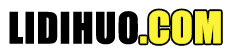# 二叉树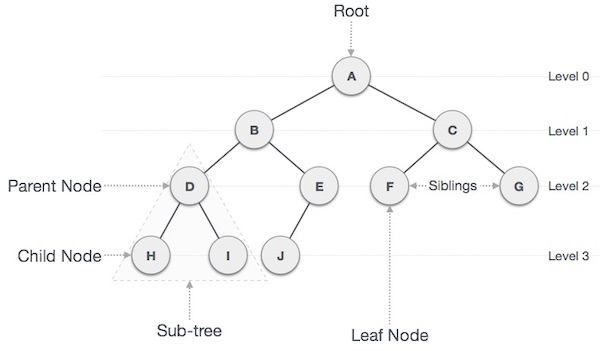### 重要条款

Path-路径是指沿树边缘的节点序列。 Root-树顶部的节点称为根。每棵树只有一个根，并且从根节点到任何节点都有一条路径。 Parent-除根节点外的任何节点都有一个向上的边缘到称为父节点的节点。 Child-给定节点下方由其边缘向下连接的节点称为其子节点。 Leaf-没有任何子节点的节点称为叶节点。 Subtree-子树代表一个节点的后代。 Visiting-访问是指当控制在节点上时检查节点的值。 Traversing-遍历意味着以特定顺序通过节点。 Levels-节点的级别表示节点的生成。如果根节点处于 0 级，则其下一个子节点处于 1 级，其孙节点处于 2 级，依此类推。 keys-键表示节点的值，根据该值对节点执行搜索操作。

### 二叉搜索树表示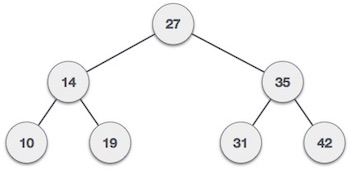### 树节点

```struct node {
int data;
struct node *leftChild;
struct node *rightChild;
};
```

### BST 基本操作

Insert-在树中插入元素/创建树。 Search-搜索树中的元素。 Preorder Traversal-以预序方式遍历树。 Inorder Traversal-以中序方式遍历树。 Postorder Traversal-以后序方式遍历树。

### 算法

```if root is null
then create root node
return
if root exists then
compare the data with node.data

while until insertion position is located
if data is greater than node.data
goto right subtree
else
goto left subtree
endwhile

insert data

end if
```

### 实施

```void insert(int data) {
struct node *tempNode = (struct node*) malloc(sizeof(struct node));
struct node *current;
struct node *parent;
tempNode->data = data;
tempNode->leftChild = null;
tempNode->rightChild = null;
//if tree is empty, create root node
if(root == null) {
root = tempNode;
} else {
current = root;
parent  = null;
while(1) {
parent = current;
//go to left of the tree
if(data < parent->data) {
current = current->leftChild;

//insert to the left
if(current == null) {
parent->leftChild = tempNode;
return;
}
}

//go to right of the tree
else {
current = current->rightChild;

//insert to the right
if(current == null) {
parent->rightChild = tempNode;
return;
}
}
}
}
}
```

### 算法

```if root.data is equal to search.data
return root
else
if data is greater than node.data
goto right subtree
else
goto left subtree

if data found
return node
endwhile

end if
```

```struct node* search(int data) {
struct node *current = root;
printf("Visiting elements: ");
while(current->data != data) {
if(current != null)
printf("%d ",current->data);

//go to left tree
if(current->data > data) {
current = current->leftChild;
}
//else go to right tree
else {
current = current->rightChild;
}
if(current == null) {
return NULL;
}
return current;
}
}
```

## 二叉树遍历

### 顺序遍历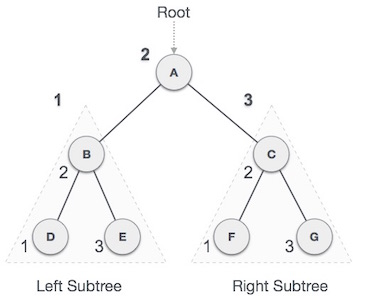D → B → E → A → F → C → G

### 算法

```Until all nodes are traversed −
Step 1 − Recursively traverse left subtree.
Step 2 − Visit root node.
Step 3 − Recursively traverse right subtree.
```

### 前序遍历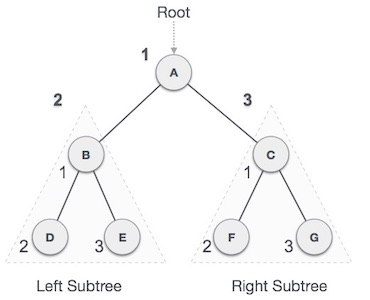A → B → D → E → C → F → G

### 算法

```Until all nodes are traversed −
Step 1 − Visit root node.
Step 2 − Recursively traverse left subtree.
Step 3 − Recursively traverse right subtree.
```

### 后序遍历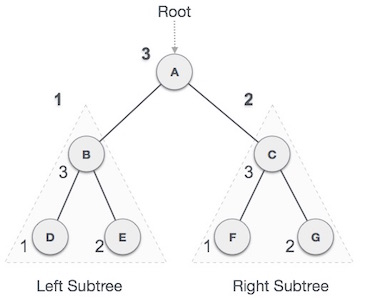D → E → B → F → G → C → A

### 算法

```Until all nodes are traversed −
Step 1 − Recursively traverse left subtree.
Step 2 − Recursively traverse right subtree.
Step 3 − Visit root node.
```

## 二叉树详细讲解

 二分搜索树 二分搜索树节点的插入 二分搜索树节点的查找 二分搜索树深度优先遍历 二分搜索树层序遍历 二分搜索树节点删除 二分搜索树的特性